## Mathematics Paper 2 Questions and Answers - Asumbi Girls Mock Examinations 2022

QUESTIONS

SECTION 1 (50 MARKS)
Answer all questions in this section.

1. Solve for x in the equation 2Sin2x - 1 = Cos2x + Sinx for 0º ≤ x ≤ 360º(3marks)
2.
1. Expand (1+3/x) 5 up to the fifth term (2marks)
2. Hence use your expansion to evaluate the value of (2.5)5 to 3 d.p. (2 marks)
3. Complete the table below for   for -4 ≤ x ≤ 2.
 x -4 -3 -2 -1 0 1 2 y
Hence use trapezium rule with six strips to find the area of the region bounded by the curve and the x- axis. (3marks)
4. Make p the subject of the formula (3 marks)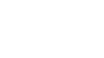5. The figure below shows a rectangular based right pyramid. Find the angle between the planes ABCD and ABV.  (3marks)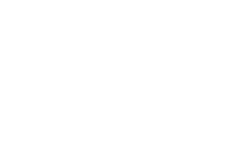6. An object A of area 10cm2 is mapped onto its image B of area 60cm2 by a transformation whose matrix is given by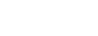. Find the possible values of x. (3 marks)
7. Find the value of x in the equation(3marks)
log105 - 2 + log1010(2x + 10) = log10(x - 4)
8. The data below shows marks obtained by 10 students in a test.
71, 55, 69, 45, 65, 57, 71, 82, 55, 50. Calculate the standard deviation using an assumed mean of 60. (3marks)
9. Evaluate by rationalizing the denominator and leaving your answer in surd form. (3marks)
√8
1 + cos45º
10. The position vectors fof points A and B are 5i + 4j - 6k and 2i - 2j respectively. A point X divides AB in the ratio -3: 5. Find the coordinates of X. (3marks)
11. A closed box has a square base of side x metres and its height h metres. The total surface area of the box is 24m2
1. Find the expression of h in terms of x.
2. Hence find the value of x that would make the volume of the box maximum. (4 marks)
12. M varies directly as D and as the cube of V. Calculate the percentage change in M when V is increased by 10% and D is reduced by 10%. (3marks)
13. Find the value of t if the gradient of the graphs of the functions y = x2 - x3 and y = x - tx2 are equal at  x = 1/3. (3marks)
14. The image of a point A, under the transformation represented by the matrix T = (10 -12) is A’ (-2, 4)Find the coordinates of A (3marks)
15. In the figure below, ABC is a tangent at B and CDE is a straight line. BED = 50º , DEF = 35º and ECB = 25º Calculate the values of x and z. (2marks)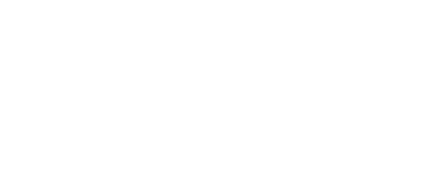16. The equation of a circle is given by  4x2 + 4y2 - 8x + 2y - 7 = 0Determine the coordinates of the centre of the circle. (3marks)

SECTION II (50 MARKS)
Answer only five questions from this section

1.
1. Using a ruler and a pair of compasses only construct triangle ABC in whichAB = 6cm, BC = 5.5cm and angle ABC = 60º. Measure AC. (3marks)
2. On the same side of AB as C, determine the locus of a point P such that angle APB = 60º (2marks)
3. Construct the locus of R such that AR = 3cm (1mark)
4. Identify the region T such that AR≥ 3cm and angleAPB≥ 60º by shading the unwanted part. (2marks)
5. Determine point Q such that area of AQB is half the area of ABC and that Angle AQB = 60º. (2marks)
2. A sequence is formed by adding corresponding terms of an AP and GP. The first, second and third terms of the sequence formed are 14, 34 and 78 respectively.
1. Given that the common ratio of the GP is 3;
1. Find the first term of the AP and GP and the common difference of the AP. (2marks)
2. Find the sixth term and the sum of the first six terms of the sequence. (3marks)
2. The second and third terms of a geometric progression are 24 and 12(x + 1) respectively.
Find the whole number value of x and hence the first term given the sum of the first three terms of the progression is 76. (5marks)
3. Income tax rate are as shown below.
 Income (k£ p.a) Rate (Ksh per £) 1- 4200 2 4201 - 8000 3 8001 - 12600 5 12601 – 16800 6 16801 and above 7
Omari pays Sh. 4000 as P.A.Y.E per month. He has a monthly house allowance of Ksh. 10800 and is entitled to a personal relief of Ksh. 1,100 per month. Determine;
1. his gross tax p.a in Ksh (2marks)
2. his taxable income in k£ p.a (4marks)
3. his basic salary in Ksh. p.m (2marks)
4. his net salary per month (2marks)
4. The diagram below shows a sketch of the line y = 3x and the curve   intersecting at point P and Q.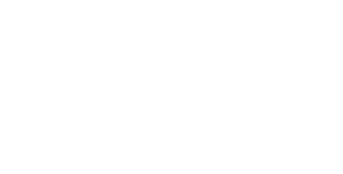1. Find the co-ordinates of P and Q (4marks)
2. Given that QN is perpendicular to the x-axis at N, calculate
1. The area bounded by the curve y = 4 - x2 , the x-axis and line QN. (2marks)
2. the area of the shaded region that lies below the x - axis (2marks)
3. the area of the region enclosed by the curve y = 4 - x2 , the line y = 3x and the y-axis (2marks)
5. The positions of two towns A and B are (50ºN, 45ºW) and (50ºN, KºW) respectively. It takes a plane 5 hours to travel from A to B at an average speed of 800knots. The same plane takes 1½ hours to travel from B to another town C at the same average speed. Given that C is to the north of B, calculate to the nearest degree,
1. The value of K (4marks)
2. The latitude of C (3marks)
3. If the plane started from A at 9.00am and flew to C through B, find the local time at C when the plane arrived there. (3marks)
6.
1. Complete the table below for the equation y = x3 + 2x2 to 2 d.p(2marks)
 x -3 -2.5 -2 -1.5 -1 -0.5 0 0.5 1 1.5 2x2 18 12.5 8 4.5 2 0 1 4.5 x3 -27 -8 -1 0 1 y -9 0 1 0 2
2. On the grid provided, draw the graph of y = x3 + 2x2 for -3 ≤ x ≤ 1.5. Take a scale of 2cm to represent 1 unit on the x- axis and 1cm to represent 1unit on the y – axis. (3marks)
3. Use your graph to solve
1. x3 + 2x2 = 0(2marks)
2. x3 + 2x2 - x - 2 = 0(3marks)
7. The table below shows the marks obtained by 47 students in a mathematics test.
 Marks 31-35 36-40 41-45 46-50 51-55 56-60 No. of candidates 4 6 12 15 8 2
1. On the grid provided, draw a cumulative frequency curve. (3marks)
2. Use your graph to estimate
1. The median (2marks)
2. The semi interquartile range (2marks)
3. In order to pass the test a candidate had to score more than 40 marks. Calculate the percentage of candidates who passed. (3marks)
8. In the triangle OPQ below, OP = p and OQ = q. R is a point on PQ such that PR: RQ = 1 : 3 and 5OS = 2 OQ. PS intersects OR at T.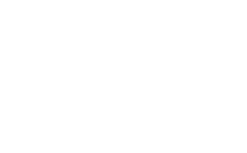1. Express in term of p and q
1. OS (1mark)
2. PQ (1mark)
3. OR (2mark)
2. Given that OT= hOR and PT = kPS. Determine the values of h and k. (6marks)## MARKING SCHEME

1. 2sin2x - 1 = 1 - sin2x + sinx
2sin2x + sin2x - sinx - 1 - 1 = 0
3sin2x - sinx - 2 = 0
3sin2x - 3sinx + 2sinx - 2 = 0
3sinx(sinx - 1) + 2(sinx - 1) = 0
(sinx - 1)(3sinx + 2) = 0
sinx - 1 = 0 or 3sinx = -2/3
3
sinx = 1      sinx = -2/3
x = 90        x = 180 + 41.81 = 138.19
x = 360 - 41.81 = 318.19
2.
1. 1 + 5(3/x) + 10(3/x)2 + 10(3/x)3 + 5(3/x)4
1 + 15/x + 90/x2 + 270/x3 + 405/x4
2. 2.5 = 1 +3/x
2.5 - 1 = 3/x
1.5 = 3/x
1.5x/1.5 = 3/15
1 + 15/2 + 90/4 + 270/8 + 405/16
= 90.0625
= 90.63

3.  x -4 -3 -2 -1 0 1 2 y 0 5 8 9 8 5 0
A = x 1{0 + 0 + 2(5 + 8 + 9 + 8 + 5)}
= x 2(35)
= 35
4. e2/1 =  p - 3u
y - 3xp
e2(y - 3xp) = p - 3u
e2y - 3e2xp = p - 3u
e2y + 3u = p + 3e2xp
p + 3e2xp = e2y + 3u
p(1 + 3e2x) = e2y + 3u
p = e2y + 3u
1 + 3e2x
5.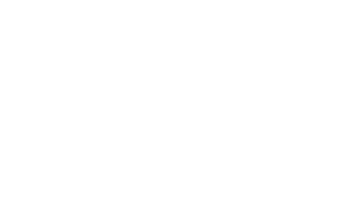6. area scale factor = 60/10 = 6
[x3 4x+3]
determinant x(x + 3) - 12 = x2 + 3x - 12
x2 + 3x - 12 = 6
- 6 = 0
- 18 = 0
+ 6x - 3x - 18 = 0
x(x + 6) -3(x + 6) = 0
(x + 6)(x - 3) = 0
x + 6 = 0         x - 3 = 0
x = -6               x=3
7. log105 - 2log1010 +log10(2x + 10) = log10(x - 4)
log105(2x + 10) - log10100 = log10(x - 4)
log10(10x + 50) = log10(x - 4)
100
10x + 50 = x - 4
100          1
10x + 50 = 100(x - 4)
10x + 50 = 100x -400
50 + 400 = 100x - 10x
450/90 = 90x/90
x = 5

8.  x x-60 (x-60)2 71 -11 121 55 -5 25 69 9 81 45 -15 225 65 5 25 57 -3 9 71 11 121 82 22 484 55 -5 25 50 -10 100 20 1216
∑(x - 2)2 - [∑(x - a)]
∑f             ∑f
√1216/10 - (20/10)2
= √ 121.6 - 4
= √117.6
= 10.84
9.      (√8)√2
(1 + 1/√2)√2
√16   =   4
√2 + 1  √2 + 1
4    √2 - 1
√2 + 1   √2 - 1
4√2 - 4
2 - 1
= 4√2 - 4
10.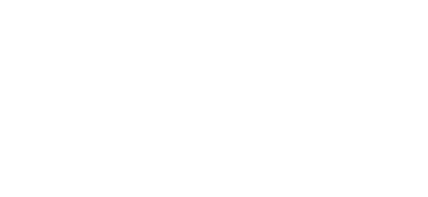11.
1. S.A = 2x2 + 4xh
2x2 + 4xh = 24
4xh = 24 - 2x2
h = 24 - 2x2
4x
h = 24/4x - 2x2 /4x
h = a/x - x/2
2. v = b.a x h
= x2 x h
= hx2
v = hx2
= x2(6/x - x/2)
v = 6x - x3/2
dv/dx = 6 - 3/2x2
6 - 3x2/2 = 0
12/3 = 3x2/3
√4 = √x2
2 = x
12. M = KDV3
M = K(0.9D)(1.1V)3
M = 0.9 x 1.13 KDV3
M = 1.1979 KDV3
= 1.1979
%change = 1.1979M - M x 100
M
= 0.1979 x 100
= 19.79%
13. y = x2 - x3
dy/dx = 2x - 3x2
y = x - tx2
dy/dx = 1 - 2tx
= 2(1/3) - 3(1/3)2
2/31/31/3
= 1 - 2t(1/3)
= 1 - 2/3t
1/3 = 1 -  2/3t
2/3t = 2/3
t = 1
14.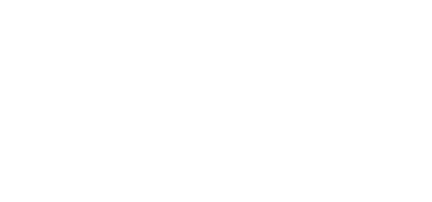15. z = 50º
x = 50 + 25
= 75º
16. 4x2/4 - 8x/4 + 4y2/4 + 2y/4 = 7/4
x2 - 2x + (-2/2)2 + y2 + (¼)27/4 + (-2/2)2 + (¼)2
(x - 1)2 + (y + )27/4 + 1 + 1/16
(x - 1)2 + = 28 + 16 + 1
16
(x - 1)2 + = 45/16
centre(1, -¼)
17.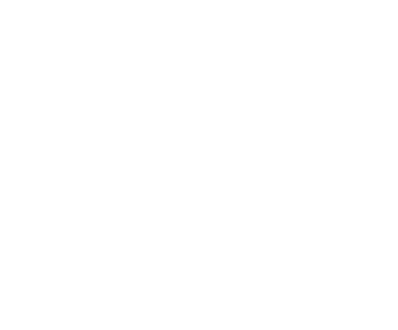Area of ΔABC = ½ x 6 x 5.5 x sin60
= 14.289
14.2894 = ½ x 6 x h
3 3
h = 4.76 ≈ 5cm
18.
1.
1. AP: a   a+d   a+2d
GP: x   xr   xr2
x   3x   9x
a + x = 14
2(a + d + 3x = 34)
a + 2d + 9x = 78
2a + 2d + 6x = 68
a + 2d + 9x = 78
a - 3x = -10
a + x = 14
-4x/-4 = -24/4
x = 6
a + x = 14
a + 6 = 14
a = 8
2. 14,34,78
T6 = a + 5d + 35x
a + d + 3x = 34
d = 34 - 26
d = 8
T6 = a + 5d + 243x
= 8 + 5(8) + 243 x 6
= 1506
T5 = a + 4d + 81x
= 8 + 4(8) + 81 x 6
= 526
T4 = a + 3d + 27x
8 + 3(8) + 27 x 6 = 194
+14 + 34 + 78 + 194 + 526 + 1506 = 2352
2. ar = 24
ar2 = 12(x + 1)
r = 12(x + 1) = x + 1
24            2
ar = 24
a(x + 1) = 24
2
a = 24 x 2 =  48
x + 1     x + 1
(48/x+1 + 24 + 12(x + 1) = 76)(x + 1)
48 + 24(x + 1) + 12(x + 1)2 = 76(x + 1)
48 + 24x + 24 + 12(x2 + 2x + 1) = 76x + 76
72 + 24x + 12x2 + 24x + 12 - 76x - 76 = 0
12x2/4 - 28x/4 + 8/4 = 0
3x2 - 7x + 2 = 0
3x2 - 6x - x + 2 = 0
3x(x - 2) -1(x -2)=0
(x - 2)(3x - 1) = 0
x = 2 or x = 1/3
x = 2
19.
1. 4000 + 1100 = 5100 x 12 = 61200
2. 4200 x 2 = 8400
3800 x 3 = 11400
4600 x 5 = 23000
= 42800
x + 6 = 18400
x = 18400 = 3066.67
6
12600 + 3066.67 = £15666.67
3. 15666.67 x 20 = 26111.11
12
- 10800 = 15311.11
4. 26111.11 - 4000 = 22111.11
20.
1. 4 - x2 = 3x
0 = x2 + 3x - 4
x2+ 4x - x - 4 = 0
x(x + 4) -1(x + 4) = 0
(x + 4)(x - 1) = 0
x = -4   x = 1
y = 3(-4)   y = 3(1)
= -12        = 3
Q(-4)   P(1,3)
2.
1. 4 - x2 = 0
(2 - x)(2 + x) = 0
x = 2    x = -2
-2-4 4 - x2dx = [4x - x3/3]-2-4
(4(-2) - (-2)3) - (4(-4) - (-4)3)
3                      3
= -5 1/3 - 51/3
= -10 2/3  = 102/3
2.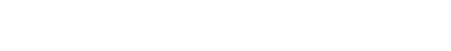3.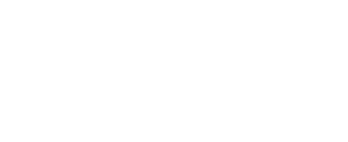21.

1.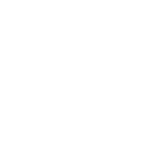D = S x T
= 800 x 5
= 4000nm
1º = 60cos50º
? = 4000
4000 x 1
60cos50
= 103.7º
k - 45 = 103.7
k = 103.7 + 45
= 148.7ºW
2. D = Sx T
= 800 x 3/2 = 1200nm
1º = 600m
? = 1200nm
1200 x 1 = 20º
60
x - 50 = 20
x = 70ºN

3.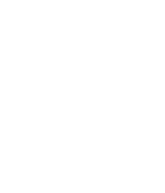Distance A - B - C
= 4000nm + 1200
= 5200nm
T = D/S
= 5200
800
= 6½hrs
θ = 103.7º
1º = 4min
103.7 = 103.7 x 4 = 414.8
60
= 6hrs55min
9:00
- 6:55
= 02.05am
+ 6:30
8:35am at C
22.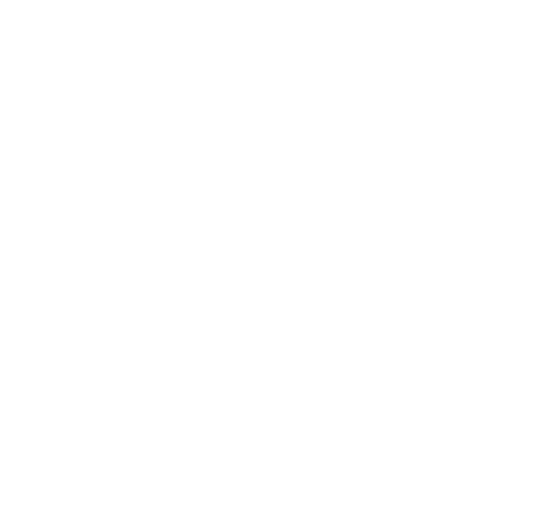23.
 Marks 31-35 36-40 41-45 46-50 51-55 56-60 No. of candidates 4 6 12 15 8 2 cf 4 10 22 37 45 47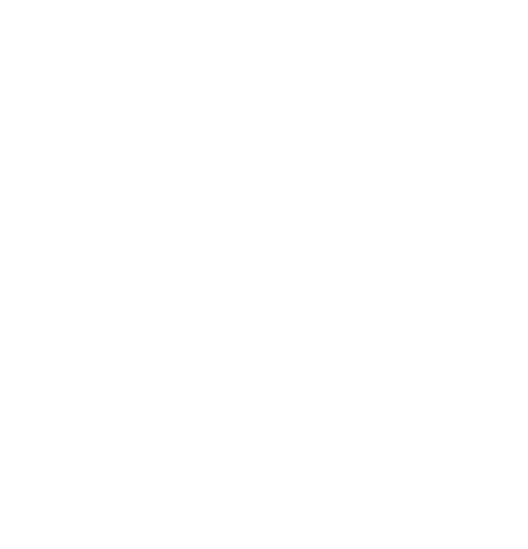24.
1.
1. 2/5OQ = 2/5q
2. q - p
3. ¼q + ¾p
2.
1. OT = h OR
= h(¼q + ¾p)
h/4q + 3h/4
2. OT = OP + PT
= p + k (-p + 2/5q)
= p - kp + 2k/5 q
= p(1-k) + 2k/5 q
h/4q + 3h/4p = p(1 - k) + 2k/5 q
3h/4 = 1 - k
4 x h/42k/5 x 4
h = 8/5k
¾(8/5k) = 1 - k
6/5k + k = 1
11/5k = 1
k = 5/11
h = 8/5
h = 8/11

• ✔ To read offline at any time.
• ✔ To Print at your convenience
• ✔ Share Easily with Friends / Students